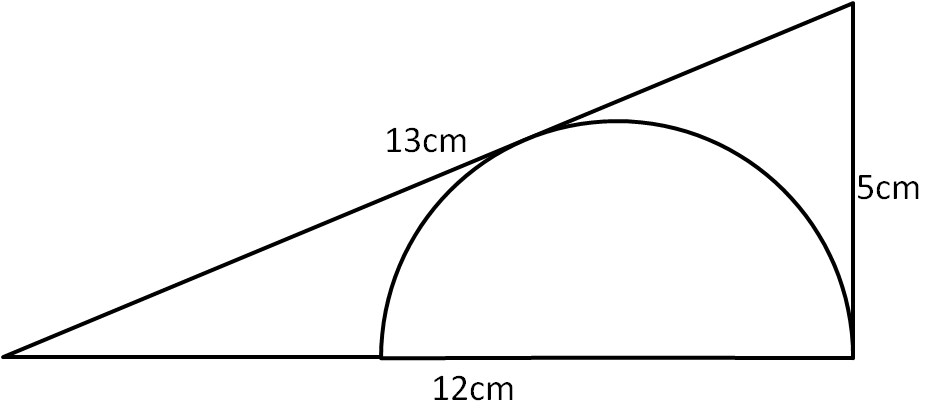You may also likeBuilding Tetrahedra

Can you make a tetrahedron whose faces all have the same perimeter?A 1 metre cube has one face on the ground and one face against a wall. A 4 metre ladder leans against the wall and just touches the cube. How high is the top of the ladder above the ground?Four rods are hinged at their ends to form a convex quadrilateral. Investigate the different shapes that the quadrilateral can take. Be patient this problem may be slow to load.

Inscribed Semicircle

Age 14 to 16 ShortChallenge Level

The diagram below shows a right angled triangle with sides of length $5\text{cm}$, $12\text{cm}$ and $13\text{cm}$. What is the radius of the inscribed semicircle whose base lies on the side of length $12\text{cm}$?If you liked this problem, here is an NRICH task that challenges you to use similar mathematical ideas.

This problem is taken from the UKMT Mathematical Challenges.
You can find more short problems, arranged by curriculum topic, in our short problems collection.